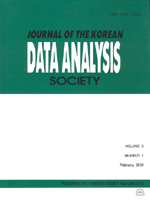상세검색
최근 검색어 전체 삭제
다국어입력
즐겨찾기0KCI등재 학술저널

# Nonparametric Tests for Multiple Endpoints with Grouped Observations

• 등재여부 : KCI등재
• 2008.02
• 75 - 86 (12 pages)

In this paper, we consider a nonparametric test procedure for the multiple endpoints with grouped data under two sample problem setting. For the construction of the test statistic, we use the linear rank statistics which were derived based on the likelihood ratio principle for each component. Then the asymptotic distribution of the test statistic is derived under the null hypothesis. Finally, we illustrate our test procedure with an example and discuss some concluding remarks.

1. Introduction

2. Multivariate nonparametric test for grouped data

3. An example

4. Some concluding remarks

References